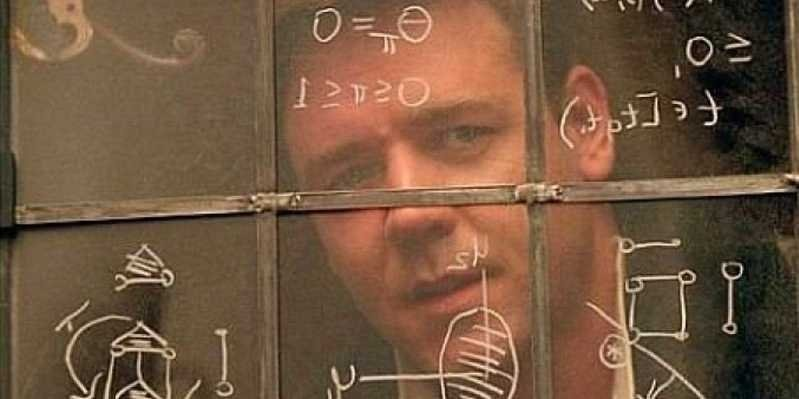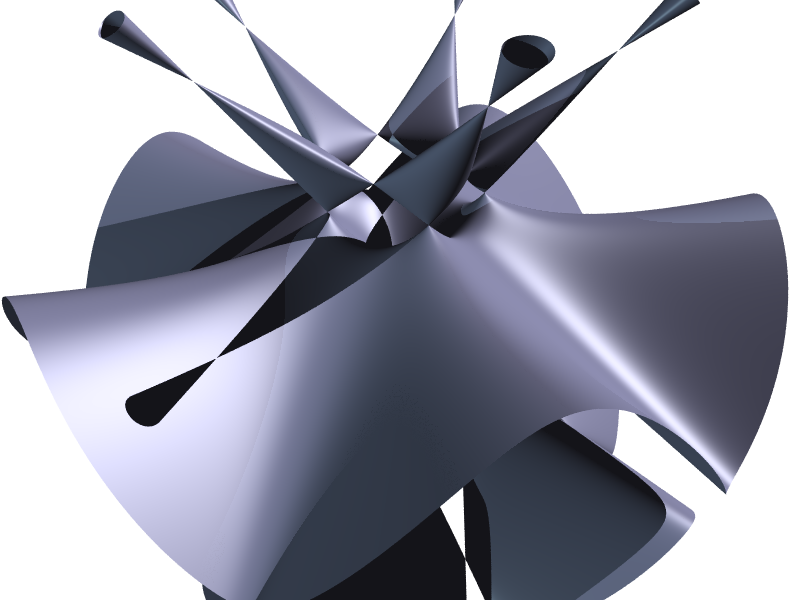In 2000, the Clay Mathematics Institute announced the Millennium Prize problems. These were a collection of seven of the most important maths problems that remain unsolved.

Reflecting the importance of the problems, the Institute offered a \$1 million (R14.7 million) prize to anyone who could provide a rigorous, peer-reviewed solution to any of the problems.

While one of the problems, the Poincare Conjecture, was famously solved in 2006 (with the mathematician who solved it, Grigori Perelman, equally famously turning down both the million dollar prize and the coveted Fields Medal), the other six problems remain unsolved.

Here are the six math problems so important that solving any one of them is worth \$1 million.

## P vs NP(Getty Images)

Some problems are easy, and some problems are hard.

In the world of maths and computer science, there are a lot of problems that we know how to program a computer to solve "quickly" — basic arithmetic, sorting a list, searching through a data table. These problems can be solved in "polynomial time," abbreviated as "P." It means the number of steps it takes to add two numbers, or to sort a list, grows manageably with the size of the numbers or the length of the list.

But there’s another group of problems for which it’s easy to check whether or not a possible solution to the problem is correct, but we don’t know how to efficiently find a solution. Finding the prime factors of a large number is such a problem — if I have a list of possible factors, I can multiply them together and see if I get back my original number. But there is no known way to quickly find the factors of an arbitrary large number. Indeed, the security of the internet relies on this fact.

For historical and technical reasons, problems where we can quickly check a possible solution are said to be solvable in “non-deterministic polynomial time,” or “NP.”

Any problem in P is automatically in NP — if I can solve a problem quickly, I can just as quickly check a possible solution simply by actually solving the problem and seeing if the answer matches my possible solution. The essence of the P vs NP question is whether or not the reverse is true: If I have an efficient way to check solutions to a problem, is there an efficient way to actually find those solutions?

Most mathematicians and computer scientists believe the answer is no. An algorithm that could solve NP problems in polynomial time would have mind-blowing implications throughout most of maths, science, and technology, and those implications are so out-of-this-world that they suggest reason to doubt that this is possible.

Of course, proving that no such algorithm exists is itself an incredibly daunting task. Being able to definitively make such a statement about these kinds of problems would likely require a much deeper understanding of the nature of information and computation than we currently have, and would almost certainly have profound and far-reaching consequences.

Read the Clay Mathematics Institute's official description of P vs NP here.

## The Navier-Stokes equations

It’s surprisingly difficult to explain what happens when you stir milk into your morning coffee.

The Navier-Stokes equations are the fluid-dynamics version of Newton’s three laws of motion. They describe how the flow of a liquid or a gas will evolve under various conditions. Just as Newton's second law gives a description of how an object's velocity will change under the influence of an outside force, the Navier-Stokes equations describe how the speed of a fluid's flow will change under internal forces like pressure and viscosity, as well as outside forces like gravity.

The Navier-Stokes equations are a system of differential equations. Differential equations describe how a particular quantity changes over time, given some initial starting conditions, and they are useful in describing all sorts of physical systems. In the case of the Navier-Stokes equations, we start with some initial fluid flow, and the differential equations describe how that flow evolves.

Solving a differential equation means finding some mathematical formula to determine what your quantity of interest actually will be at any particular time, based on the equations that describe how the quantity changes. Many physical systems described by differential equations, like a vibrating guitar string, or the flow of heat from a hot object to a cold object, have well-known solutions of this type. The Navier-Stokes equations, however, are harder. Mathematically, the tools used to solve other differential equations have not proven as useful here. Physically, fluids can exhibit chaotic and turbulent behavior: Smoke coming off a candle or cigarette tends to initially flow smoothly and predictably, but quickly devolves into unpredictable vortices and whorls.

It's possible that this kind of turbulent and chaotic behaviour means that the Navier-Stokes equations can't actually be solved exactly in all cases. It might be possible to construct some idealized mathematical fluid that, following the equations, eventually becomes infinitely turbulent.

Anyone who can construct a way to solve the Navier-Stokes equations in all cases, or show an example where the equations cannot be solved, would win the Millennium Prize for this problem.

## Yang-Mills theory and the quantum mass gapBernhard Riemann
Public Doman

Maths and physics have always had a mutually beneficial relationship. Developments in mathematics have often opened new approaches to physical theory, and new discoveries in physics spur deeper investigations into their underlying mathematical explanations.

Quantum mechanics has been, arguably, the most successful physical theory in history. Matter and energy behave very differently at the scale of atoms and subatomic particles, and one of the great achievements of the 20th century was developing a theoretical and experimental understanding of that behaviour.

One of the major underpinnings of modern quantum mechanics is Yang-Mills theory, which describes the quantum behaviour of electromagnetism and the weak and strong nuclear forces in terms of mathematical structures that arise in studying geometric symmetries. The predictions of Yang-Mills theory have been verified by countless experiments, and the theory is an important part of our understanding of how atoms are put together.

Despite that physical success, the theoretical mathematical underpinnings of the theory remain unclear. One particular problem of interest is the "mass gap," which requires that certain subatomic particles that are in some ways analogous to massless photons instead actually have a positive mass. The mass gap is an important part of why nuclear forces are extremely strong relative to electromagnetism and gravity, but have extremely short ranges.

The Millennium Prize problem, then, is to show a general mathematical theory behind the physical Yang-Mills theory, and to have a good mathematical explanation for the mass gap.

## The Riemann HypothesisWikimedia Commons

Going back to ancient times, the prime numbers — numbers divisible only by themselves and 1 — have been an object of fascination to mathematicians. On a fundamental level, the primes are the "building blocks" of the whole numbers, as any whole number can be uniquely broken down into a product of prime numbers.

Given the centrality of the prime numbers to mathematics, questions about how primes are distributed along the number line — that is, how far away prime numbers are from each other — are active areas of interest.

By the 19th century, mathematicians had discovered various formulas that give an approximate idea of the average distance between primes. What remains unknown, however, is how close to that average the true distribution of primes stays — that is, whether there are parts of the number line where there are "too many" or "too few" primes according to those average formulas.

The Riemann Hypothesis limits that possibility by establishing bounds on how far from average the distribution of prime numbers can stray. The hypothesis is equivalent to, and usually stated in terms of, whether or not the solutions to an equation based on a mathematical construct called the "Riemann zeta function" all lie along a particular line in the complex number plane. Indeed, the study of functions like the zeta function has become its own area of mathematical interest, making the Riemann Hypothesis and related problems all the more important.

Like several of the Millennium Prize problems, there is significant evidence suggesting that the Riemann Hypothesis is true, but a rigorous proof remains elusive. To date, computational methods have found that around 10 trillion solutions to the zeta function equation fall along the required line, with no counter-examples found.

Of course, from a mathematical perspective, 10 trillion examples of a hypothesis being true absolutely does not substitute for a full proof of that hypothesis, leaving the Riemann Hypothesis one of the open Millennium Prize problems.

## The Birch and Swinnerton-Dyer conjecture

One of the oldest and broadest objects of mathematical study are the diophantine equations, or polynomial equations for which we want to find whole-number solutions. A classic example many might remember from high school geometry are the Pythagorean triples, or sets of three integers that satisfy the Pythagorean theorem x+ y= z2.

In recent years, algebraists have particularly studied elliptic curves, which are defined by a particular type of diophantine equation. These curves have important applications in number theory and cryptography, and finding whole-number or rational solutions to them is a major area of study.

One of the most stunning mathematical developments of the last few decades was Andrew Wiles' proof of the classic Fermat's Last Theorem, stating that higher-power versions of Pythagorean triples don't exist. Wiles' proof of that theorem was a consequence of a broader development of the theory of elliptic curves.

The Birch and Swinnerton-Dyer conjecture provides an extra set of analytical tools in understanding the solutions to equations defined by elliptic curves.

## The Hodge conjectureClaudio Rocchini via Wikimedia Commons

The mathematical discipline of algebraic geometry is, broadly speaking, the study of the higher-dimensional shapes that can be defined algebraically as the solution sets to algebraic equations.

As an extremely simple example, you may recall from high school algebra that the equation y = x2 results in a parabolic curve when the solutions to that equation are drawn out on a piece of graph paper. Algebraic geometry deals with the higher-dimensional analogues of that kind of curve when one considers systems of multiple equations, equations with more variables, and equations over the complex number plane, rather than the real numbers.

The 20th century saw a flourishing of sophisticated techniques to understand the curves, surfaces, and hyper-surfaces that are the subjects of algebraic geometry. The difficult-to-imagine shapes can be made more tractable through complicated computational tools.

The Hodge conjecture suggests that certain types of geometric structures have a particularly useful algebraic counterpart that can be used to better study and classify these shapes.